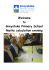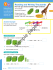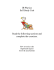# 4 Area of Squares and Rectangles

## Transcription

4 Area of Squares and Rectangles
```4
Flash Cards ÷7 and ×10
Speed Drill 4, page 62
Area of Squares and Rectangles
To find the area of a square or rectangle, multiply the length by the width.
length
4 cm
Area = 4 cm × 2 cm = 8 cm
width
3 cm
width
2 cm
length
AREA = length × width
3 cm
Area = 3 cm × 3 cm = 9 cm2
2
Write a multiplication sentence for each picture. Give the area in square feet (ft ).
One is done for you.
2 ft
5 ft
4 ft
2
1. a.
5 ft
6 ft
3 ft
4 × 3 = 12 ft2
b.
c.
Write a multiplication sentence and give the area in square yards.
14
2.
length, 7 yards
width, 6 yards
b.
length, 10 yards
width, 10 yards
c.
length, 8 yards
width, 2 yards
Lesson 4
Looking for Addends That Equal 10
We can add addends in any order as long as we don’t skip any. It may be easier to add
long columns of numbers if we can find sets of addends that equal 10 and add them first.
9
5
1
6
2
+2
9 + 1 = 10, 6 + 2 + 2 = 10
Two tens equal 20
5 is left. 20 + 5 = 25
59
64
31
11
3. a. + 2 0
2
3
5
1
b. + 6
9
3
1
7
c. + 4
7
2
4
6
8
1
6
4
3
d. + 5
10
10
5
7
5
4
3
e. + 5
2
8
4
1
5
W e R emember
Write the name of each solid.
4. a.
b.
c
d.
Round to the nearest 100 to estimate.
5. a. estimate 459 – 325
–
=
b. estimate 781 + 123
+
=
e.
c. estimate 987 – 321
–
=
15
Lesson 4
6. Rachel spends 90 minutes a day talking to
her teacher and the 8 other students in her
class over the two-way radio on the School
of the Air. Rachel works on school studies 5
days a week. How many minutes
does she spend talking with her
teacher each week?
7. If Rachel spent
and another
3
6
4
6
My Work Space
of an hour doing math,
My Work Space
of an hour studying geogra-
phy, how much more time did she spend
doing math than geography?
Write the number. Watch for zeros.
8. Forty-two million, seventy-eight thousand, three hundred twenty-one
Circle the numbers that have 3 as a factor.
Underline those that also have 9 as a factor.
9. 297
142
90
378
Circle the numbers that have 2 as a factor.
Underline the numbers that have 5 as a factor.
Put an X on the numbers that have 10 as a factor.
10. 20
16
45
72
120
504
145
57
86
49
11. a. × 7 0
37
b. × 2 0
Check
12. a.
3
4
5
7
+1
8
6
2
4
9
b.
63
×2 4
1
÷
21
c. × 4 0
×
3
1,083
d. –
99
Check
–
4
49
f. × 8
9,301
e. – 2 , 8 4 1
c. 3 ) 5 2
b. 32 ÷ 5 =
5
Check
Copy the division problems in the division boxes. Divide.
13. a. 24 ÷ 7 =
<
¥
S kill B uilders +÷ x
2
Lesson 4
Check
c. 69 ÷ 3 =
Measure to the nearest centimeter.
14.
Circle the best estimate.
15. A pair of boots
16. A turkey
2g
5g
2 kg
5 kg
200 kg
500 kg
17
Lessons 4, 5
M ental M ath . . . ?
17. a. 632,000 ÷ 100 =
18. a. 2,100 ÷ 10 =
b. 20 × 1,000 =
c. 6,000 ÷ 1,000 =
b. 72 × 10 =
c. 356 × 100 =
Write the numbers.
19.
is a factor of any even number.
21.
is a factor of any number that ends in 5 or 0.
20.
is a factor of any number that ends in 0.
Name the triangle.
22.
W
U
V
5
Benjamin
Banneker was a
free African American
who lived in the 1700s.
With only four years of grammar school, Benjamin taught
himself astronomy and how to
survey land. He learned so much
math that he was able to carve a
working clock out of wood, write
an almanac, and help survey
the land upon which the
capital city of
Washington, D.C.
was built.
Flash Cards ÷9 and Set C
18
Speed Drill 5, page 63
Lesson 5
J ust for F un
Interesting Timepieces
What tool do we use to measure time?
But this is not the only way.
A clock or a watch.
Here are some interesting ways to measure time.
Hourglass
Sundial
Sun’s Position
Sand falls into the
bottom at the same
speed every time you
flip the glass.
The sun casts its
same place on the
dial at the same time
every day.
The sun is at nearly
the same place in the
sky at the same time
every day.
1. What timepiece did God use to give a sign that Hezekiah would be healed?
19
6
Flash Cards ÷6 and ×5
Speed Drill 6, page 63
Use Digit Sums to Check Multiplication
Follow the steps to check multiplication using digit sums.
Step 1
Find the digit sum of each factor. Circle each digit sum to help you keep track
of it. (8 and 8)
Step 2
134 8
× 98 8 1
1,0 7 2
1 2,0 6 0
1 3,1 3 2 1
Step 3
Step 4
Multiply the digit sums of the factors
(8 × 8 = 64). Find the digit sum of the
answer. (6 + 4 = 10; 1 + 0 = 1) The
digit sum is 1.
Find the digit sum of the product.
(1 + 3 + 1 + 3 + 2 = 10; 1 + 0 = 1)
Compare digit sums from Step 2 and
Step 3 (1 = 1).
If the digit sums are the same, your
you did not check correctly.
Find the products. Check by comparing digit sums.
1. a.
37
×15
b.
52
×48
2. a.
20
3. a.
b.
b.
c.
c.
c.
61
×73
Lesson 6
W e R emember
Write the number for the point marked by the arrow for each scale.
0
10
4. a.
0
2
4
6
8
10
b.
Write these numbers on the blanks. Write zeros in the empty places.
5. Twelve million, five hundred eight
,
,
7. Seven hundred million,
one hundred twenty-four thousand
,
,
6. Twenty-two million
,
,
8. Alice Springs usually gets about 6 inches of
rain a year. But one day in January 1986,
Whim Creek received 5 times that much
rain all in one day! How much rain did
Whim Creek receive that January day?
My Work Space
9. In Australia’s vast, dry grasslands, jumbuck
stations are very large. The station owned
by Jason and Rachel’s dad is 50 kilometers
long and 35 kilometers wide. What is the
area of this station?
Remember: Area = length × width
My Work Space
21
Lesson 6
Check
419
327
10. a. + 7 1
11. a.
+
Check
5,009
280
b. –
7
16
9
16
b.
76
×3 2
1
÷
×
27
c. × 4 0
3
6
1
5
7
3
d. + 4
Check
–
4
<
Look for
that equal
10.
5
2
3
7
1
e. + 4
c. 2 ) 9 3
¥
S kill B uilders +÷ x
2
.
.
.
.
.
1
5
8
4
1
456
3
f. ×
Check
Count by 12’s to 144.
12.
Write the numbers.
13.
14.
is a factor of any number with a digit sum of 3, 6, or 9.
is a factor of any number with a digit sum of 9.
Measure the sides of the rectangle in inches.
15. FG is
16. GH is
inch(es)
inch(es)
F
G
I
H
17. Write an addition sentence and find the perimeter.
18. Write a multiplication sentence and find the area.
22
=
=
Lesson 6
Name the parts of a division problem.
19. 63 is the
.
21. 3 is the
.
20. 21 is the
21
)
3 63
.
B
22. Name the line.
L
G
23. Name the line segment.
4
1
b. 5 6 =
25.
26.
27.
28.
2
c. 7 3 =
Match by writing the decimals beside the words.
9.36
M
C
Change these mixed numbers to improper fractions.
24. a. 2 7 =
Swans
have
25,000
feathers.
63.9
nine and thirty-six hundredths
52.07
1
d. 5 9 =
25.70
twenty-five and seventy-hundredths
fifty-two and seven-hundredths
sixty-three and nine-tenths
Measure the gecko to the fourth-inch.
29. The gecko is
inches long.
23
```

### Dukono - Carlyle Collective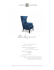### MasterCard My Account Registration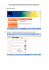### International Order Form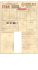### LOOKING AT LABELS Take any food item, look at its label and paste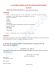### How to...view monthly pay statements and schedules (V0.3) 01.2016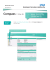### Large 2 digit BCD input 1/8 DIN display - Model BCD-2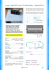### Year 5 Autumn Term Planning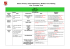### Round 4-Digit Numbers 3-digit numbers to help you round 4-digit numbers.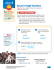### Round 2-Digit and 3-Digit Numbers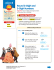### Gifts That Keep On Giving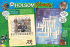### Titleist Loyalty Rewarded - Rolling Meadows Golf Course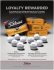### dom of aug2012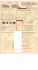### Year 4 Spring Term Planning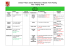### 79¢ \$1.49 - Carpinito Brothers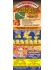### Parents Evening Written Calculation Methods